x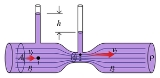Encyclopedia
Pressure head is a term used in fluid mechanics
Fluid mechanics
Fluid mechanics is the study of fluids and the forces on them. Fluid mechanics can be divided into fluid statics, the study of fluids at rest; fluid kinematics, the study of fluids in motion; and fluid dynamics, the study of the effect of forces on fluid motion...

to represent the internal energy
Internal energy
In thermodynamics, the internal energy is the total energy contained by a thermodynamic system. It is the energy needed to create the system, but excludes the energy to displace the system's surroundings, any energy associated with a move as a whole, or due to external force fields. Internal...

of a fluid
Fluid
In physics, a fluid is a substance that continually deforms under an applied shear stress. Fluids are a subset of the phases of matter and include liquids, gases, plasmas and, to some extent, plastic solids....

due to the pressure
Pressure
Pressure is the force per unit area applied in a direction perpendicular to the surface of an object. Gauge pressure is the pressure relative to the local atmospheric or ambient pressure.- Definition :...

exerted on its container. It may also be called static pressure head or simply static head (but not static head pressure). It is mathematically expressed as: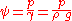whereis pressure head (Length
Length
In geometric measurements, length most commonly refers to the longest dimension of an object.In certain contexts, the term "length" is reserved for a certain dimension of an object along which the length is measured. For example it is possible to cut a length of a wire which is shorter than wire...

, typically in units of m);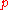is fluid pressure
Pressure
Pressure is the force per unit area applied in a direction perpendicular to the surface of an object. Gauge pressure is the pressure relative to the local atmospheric or ambient pressure.- Definition :...

(force
Force
In physics, a force is any influence that causes an object to undergo a change in speed, a change in direction, or a change in shape. In other words, a force is that which can cause an object with mass to change its velocity , i.e., to accelerate, or which can cause a flexible object to deform...

per unit area
Area
Area is a quantity that expresses the extent of a two-dimensional surface or shape in the plane. Area can be understood as the amount of material with a given thickness that would be necessary to fashion a model of the shape, or the amount of paint necessary to cover the surface with a single coat...

, often as Pa
Pascal (unit)
The pascal is the SI derived unit of pressure, internal pressure, stress, Young's modulus and tensile strength, named after the French mathematician, physicist, inventor, writer, and philosopher Blaise Pascal. It is a measure of force per unit area, defined as one newton per square metre...

units); andis the specific weight
Specific weight
The specific weight is the weight per unit volume of a material. The symbol of specific weight is γ ....

(force
Force
In physics, a force is any influence that causes an object to undergo a change in speed, a change in direction, or a change in shape. In other words, a force is that which can cause an object with mass to change its velocity , i.e., to accelerate, or which can cause a flexible object to deform...

per unit volume
Volume
Volume is the quantity of three-dimensional space enclosed by some closed boundary, for example, the space that a substance or shape occupies or contains....

, typically N/m3 units)is the density
Density
The mass density or density of a material is defined as its mass per unit volume. The symbol most often used for density is ρ . In some cases , density is also defined as its weight per unit volume; although, this quantity is more properly called specific weight...

of the fluid (mass
Mass
Mass can be defined as a quantitive measure of the resistance an object has to change in its velocity.In physics, mass commonly refers to any of the following three properties of matter, which have been shown experimentally to be equivalent:...

per unit volume
Volume
Volume is the quantity of three-dimensional space enclosed by some closed boundary, for example, the space that a substance or shape occupies or contains....

, typically kg/m3)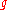is acceleration due to gravity
Standard gravity
Standard gravity, or standard acceleration due to free fall, usually denoted by g0 or gn, is the nominal acceleration of an object in a vacuum near the surface of the Earth. It is defined as precisely , or about...

(rate of change of velocity, given in m/s2)

## Practical uses for pressure head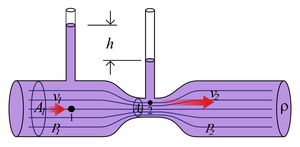Fluid flow is measured
Flow measurement
Flow measurement is the quantification of bulk fluid movement. Flow can be measured in a variety of ways.Positive-displacement flow meters acumulate a fixed volume of fluid and then count the number of times the volume is filled to measure flow...

with a wide variety of instruments. The venturi meter in the diagram on the left shows two columns of a measurement fluid at different heights. The height of each column of fluid is proportional to the pressure of the fluid. To demonstrate a classical measurement of pressure head, we could hypothetically replace the working fluid
Working fluid
A working fluid is a pressurized gas or liquid that actuates a machine. Examples include steam in a steam engine, air in a hot air engine and hydraulic fluid in a hydraulic motor or hydraulic cylinder...

with another fluid having different physical properties.

For example, if the original fluid were water
Water
Water is a chemical substance with the chemical formula H2O. A water molecule contains one oxygen and two hydrogen atoms connected by covalent bonds. Water is a liquid at ambient conditions, but it often co-exists on Earth with its solid state, ice, and gaseous state . Water also exists in a...

and we replaced it with mercury
Mercury (element)
Mercury is a chemical element with the symbol Hg and atomic number 80. It is also known as quicksilver or hydrargyrum...

at the same pressure, we would expect to see a rather different value for pressure head. In fact, the specific weight
Specific weight
The specific weight is the weight per unit volume of a material. The symbol of specific weight is γ ....

of water is 9.8 kN/m3 and the specific weight of mercury is 133 kN/m3. So, for any particular measurement of pressure head, the height of the column of water will be about 13.6 times taller than the column of mercury would be (133/9.8 = 13.6). So if a water column meter reads "13.6 cm H2O," then an equivalent measurement is "1.00 cm Hg."

This example demonstrates why there is some confusion surrounding pressure head and its relationship to pressure. Scientists frequently use columns of water (or mercury) to measure pressure, since for a given fluid, pressure head is proportional to pressure. Measuring pressure in units of "mm of mercury" or "inches of water" makes sense for instrumentation
Instrumentation
Instrumentation is defined as the art and science of measurement and control of process variables within a production, or manufacturing area....

, but these raw measurements of head must frequently be converted to more convenient pressure units using the equations above to solve for pressure.

In summary, pressure head is a measurement of length, which can be converted to the units of pressure, as long as strict attention is paid to the density of the measurement fluid and the local value of g.

## Implications for gravitational anomalies onWe would normally use pressure head calculations in areas in whichis constant. However, if the gravitational field fluctuates, we can prove that pressure head fluctuates with it.
• If we consider what would happens as gravity decreases, we would expect the fluid in the venturi meter shown above to withdraw from the pipe up into the vertical columns. Pressure head is increased.
• In the case of weightlessness
Weightlessness
Weightlessness is the condition that exists for an object or person when they experience little or no acceleration except the acceleration that defines their inertial trajectory, or the trajectory of pure free-fall...

, the pressure head approaches infinity
Infinity
Infinity is a concept in many fields, most predominantly mathematics and physics, that refers to a quantity without bound or end. People have developed various ideas throughout history about the nature of infinity...

. Fluid in the pipe may "leak out" of the top of the vertical columns (assuming).
• To simulate negative gravity, we could turn the venturi meter shown above upside down. In this case gravity is negative, and we would expect the fluid in the pipe to "pour out" the vertical columns. Pressure head is negative (assuming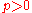).
• Ifand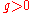, we observe that the pressure head is also negative, and the ambient air is sucked into the columns shown in the venturi meter above. This is called a siphon
Siphon
The word siphon is sometimes used to refer to a wide variety of devices that involve the flow of liquids through tubes. But in the English language today, the word siphon usually refers to a tube in an inverted U shape which causes a liquid to flow uphill, above the surface of the reservoir,...

, and is caused by a partial vacuum
Vacuum
In everyday usage, vacuum is a volume of space that is essentially empty of matter, such that its gaseous pressure is much less than atmospheric pressure. The word comes from the Latin term for "empty". A perfect vacuum would be one with no particles in it at all, which is impossible to achieve in...

inside the vertical columns. In many venturis, the column on the left has fluid in it (), while only the column on the right is a siphon (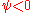).
• If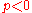and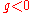, we observe that the pressure head is again positive, predicting that the venturi meter shown above would look the same, only upside down. In this situation, gravity causes the working fluid to plug the siphon holes, but the fluid doesn't leak out because the ambient pressure is greater than the pressure in the pipe.
• The above situations imply that the Bernoulli equation
Bernoulli's principle
In fluid dynamics, Bernoulli's principle states that for an inviscid flow, an increase in the speed of the fluid occurs simultaneously with a decrease in pressure or a decrease in the fluid's potential energy...

, from which we obtain static pressure head, is extremely versatile.

## Applications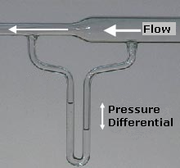### Static

A mercury barometer is one of the classic uses of static pressure head. Such barometers are an enclosed column of mercury, designed to be open to the atmosphere to measure atmospheric pressure
Atmospheric pressure
Atmospheric pressure is the force per unit area exerted into a surface by the weight of air above that surface in the atmosphere of Earth . In most circumstances atmospheric pressure is closely approximated by the hydrostatic pressure caused by the weight of air above the measurement point...

. The reading of a mercury
Mercury (element)
Mercury is a chemical element with the symbol Hg and atomic number 80. It is also known as quicksilver or hydrargyrum...

barometer (in mm of Hg
Torr
The torr is a non-SI unit of pressure with the ratio of 760 to 1 standard atmosphere, chosen to be roughly equal to the fluid pressure exerted by a millimetre of mercury, i.e., a pressure of 1 torr is approximately equal to 1 mmHg...

, for example) can be converted into an absolute pressure using the above equations.

If we had a column of mercury 767 mm high, we could calculate the atmospheric pressure as (767 mm)•(133 kN/m³) = 102 kPa. See the torr and pascal (unit)
Pascal (unit)
The pascal is the SI derived unit of pressure, internal pressure, stress, Young's modulus and tensile strength, named after the French mathematician, physicist, inventor, writer, and philosopher Blaise Pascal. It is a measure of force per unit area, defined as one newton per square metre...

articles for barometric pressure measurements at standard conditions.

### Differential

The venturi meter and manometer (shown on the right) is a common type of flow meter which can be used in many liquid applications to convert differential pressure heads into volumetric flow rate
Volumetric flow rate
The volumetric flow rate in fluid dynamics and hydrometry, is the volume of fluid which passes through a given surface per unit time...

, linear fluid speed
Speed
In kinematics, the speed of an object is the magnitude of its velocity ; it is thus a scalar quantity. The average speed of an object in an interval of time is the distance traveled by the object divided by the duration of the interval; the instantaneous speed is the limit of the average speed as...

, or mass flow rate
Mass flow rate
Mass flow rate is the mass of substance which passes through a given surface per unit time. Its unit is mass divided by time, so kilogram per second in SI units, and slug per second or pound per second in US customary units...

using Bernoulli's principle
Bernoulli's principle
In fluid dynamics, Bernoulli's principle states that for an inviscid flow, an increase in the speed of the fluid occurs simultaneously with a decrease in pressure or a decrease in the fluid's potential energy...

. The reading of these meters (in inches of water, for example) can be converted into a differential, or gauge pressure, using the above equations.

• Pressure measurement
Pressure measurement
Many techniques have been developed for the measurement of pressure and vacuum. Instruments used to measure pressure are called pressure gauges or vacuum gauges....

• Derivations of Bernoulli equation